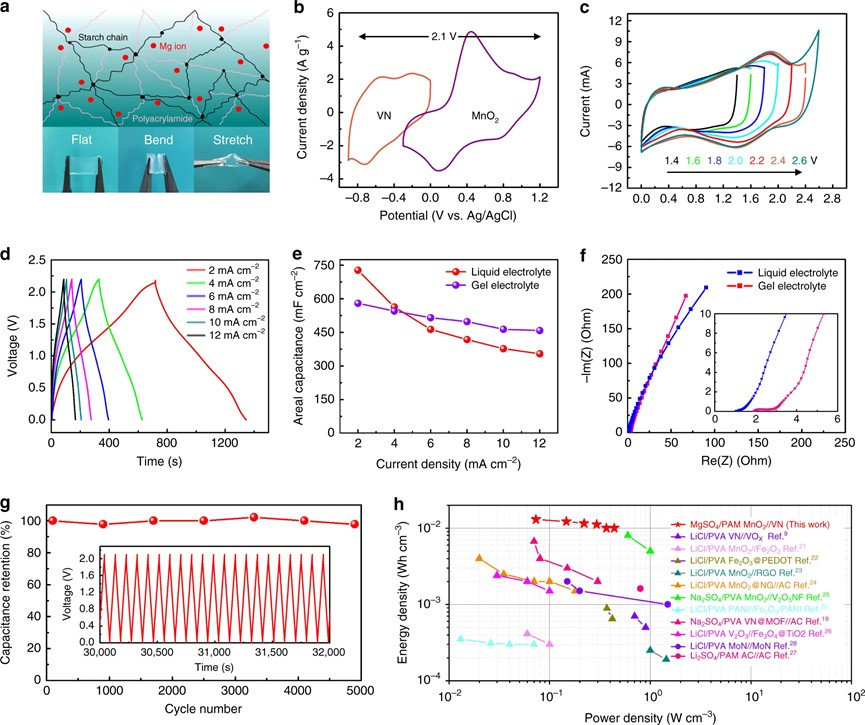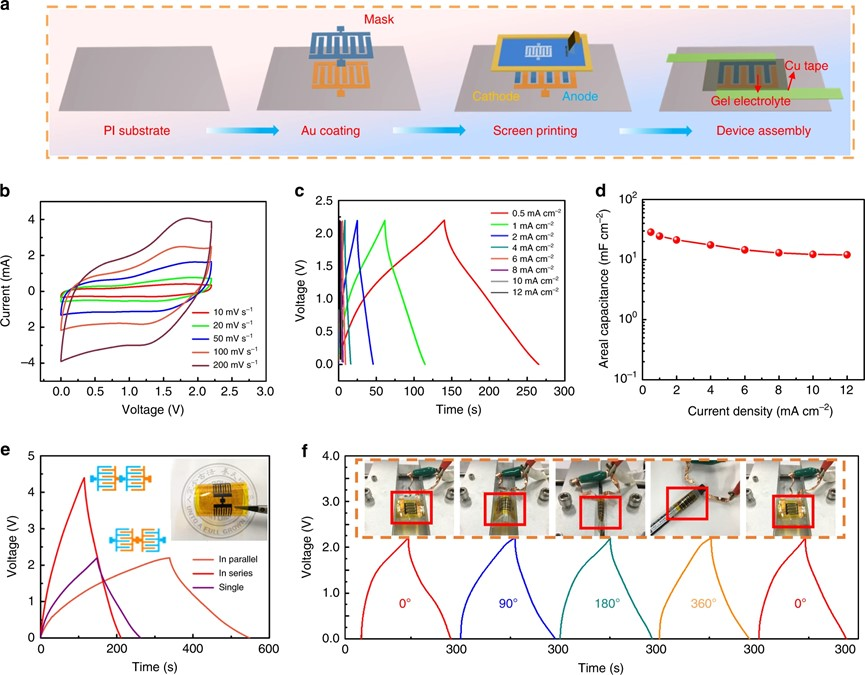# 苏大孙靖宇&邵元龙NC.：印刷的镁离子基超级电容器用于可穿戴太阳能自供电单元Fig. 1 Schematic illustration of the solar-charging integrated unit and characterization of VN nanowires. (a) Configuration of the solar-charging self-powered unit and proof-of-concept demonstration of wearable applications. (b) SEM image of as-prepared porous VN nanowires. (c) TEM image of VN nanowires. Inset: High-resolution STEM image. (d) XRD pattern of VN.Fig. 2 Electrochemical characterization of VN in a three-electrode configuration. (a) CV curves measured in different cation-based electrolytes at the same scan rate of 10 mV s−1. (b) Corresponding gravimetric specific capacitances calculated from a in different electrolytes. (c) Polarization curves of a VN electrode in different cation-based neutral electrolytes. (d) Specific capacitances of VN in 1.0 M MgSO4 electrolyte at different scan rates. Inset: Corresponding CV curves at different scan rates. (e) Capacitive and diffusion-controlled contributions to the total charge storage of VN in 1.0 M MgSO4 at 10 mV s−1. (f) Normalized contribution ratio of capacitive (cyan) and diffusion-controlled (orange) capacities at different scan rates.Fig. 3 Electrochemical reaction mechanism of VN in a neutral MgSO4 electrolyte. (a) Operando XRD patterns of VN during the charge/discharge process (current density: 0.1 A g−1). (b) Schematic diagram showing the contraction and expansion of the (200) crystal plane during the charge/discharge process. (c) Ex situ XPS Mg 1s spectra at different potentials during the CV scan at a scan rate of 5 mV s−1. (d) Change of vanadium valence state according to XPS V 2p spectra.Fig. 4 Electrochemical performance of Mg ion quasi-solid-state ASCs. (a) Structural diagram of a PAM gel electrolyte and digital photos showing its mechanical robustness upon deformation. (b) CV curve obtained for the VN and MnO2 electrodes, respectively, at different potential ranges at a scan rate of 10 mV s−1. (c) CV curves of a quasi-solid-state ASC with an increasing voltage window from 1.4 to 2.6 V at 10 mV s−1. (d) Galvanostatic charge/discharge curves of ASCs under different current densities. (e) Comparison of areal specific capacitance of a liquid electrolyte and a gel electrolyte under different current densities. (f ) Nyquist plot of a liquid electrolyte/separator and a gel electrolyte. (g) Long-term cycling stability of a quasi-solid-state ASC at a charge/discharge current density of 16 mA cm−2. (h) Ragone plot of our quasi-solid-state ASC devices in comparison with other recently reported quasi-solid-state ASCs in neutral electrolytes.Fig. 5 Electrochemical performance of a flexible and printable quasi-solid-state MASC. (a) Schematic illustration of the fabrication process of a MgSO4/PAM VN//MnO2 MASC. (b) CV curves of a printed MASC obtained at different scan rates. (c) Galvanostatic charge/discharge curves tested at different current densities. (d) Calculated areal specific capacitance at different current densities corresponding to c. (e) Galvanostatic charge/discharge curves obtained at 0.5 mA cm−2 of single, series and parallel connections of two MASCs. (f) Galvanostatic charge/discharge curves tested at 0.5 mA cm−2 under different bending angles.Fig. 6 Electrochemical performance of as-constructed solar-charging integrated units. (a) Solar-charging/discharging curves at different light intensities and the same discharge current density of 1 mA cm−2. (b) Calculated ηoverall at different light intensities corresponding to a. (c) Comparison of ηoverall with a recently reported solar-charging integrated energy system. (d) Voltage–time profiles of our flexible solar-charging integrated units. (e) Solar-charging/discharging curves at different bending angles (left panel), with digital photos (right panel) showing bending conditions. (f) Durability test of a flexible solar charging integrated unit for 100 cycles. (g) Long cycling stability of a flexible solar-charging integrated unit. (h) Demonstration of wearable scenarios using our solar-charging integrated units.